# Half-life (element) facts for kids

Kids Encyclopedia FactsSimulation of many identical atoms undergoing radioactive decay, starting with either 4 atoms per box (left) or 400 (right). The number at the top is how many half-lives have elapsed. Note the law of large numbers: With more atoms, the overall decay is more regular and more predictable.
Number of
half-lives
that have happened
Parts
remaining
As
power
of 2
0 1/1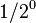$1/2^0$
1 1/2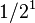$1/2^1$
2 1/4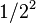$1/2^2$
3 1/8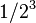$1/2^3$
4 1/16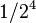$1/2^4$
5 1/32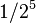$1/2^5$
6 1/64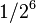$1/2^6$
7 1/128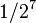$1/2^7$
... ...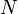$N$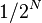$1/2^N$$1/2^N$

The half-life of a substance is the time it takes for half of the substance to decay. The word "half-life" was first used when talking about radioactive elements where the number of atoms get smaller over time. It is now used in many other situations where something declines exponentially. A Geiger-Muller detector can be used to measure the half-life; it is the time when the activity is half of the original.

Note that half-life is defined as a probability. Half-life is the expected value when half the number of atoms have decayed. Carbon-14 has a half-life of 5,730 years. Taking one atom of 14C, this will either have decayed after 5,730 years, or it will not. But if this experiment is repeated again and again, it will be seen that the atom decays within the half life 50% of the time.

Radioactive atoms have unstable nuclei. Their nuclei are unstable because the arrangement of protons and neutrons in them are unsteady. This makes them shake until they begin to decay and change into completely different types of atoms by releasing certain types of radioactive particles, such as alpha particles, beta particles or gamma rays, and possibly by fissioning into smaller nuclei. This is known as radioactive decay. For example, a radioactive carbon-14 atom releases a beta particle to become nitrogen-14. As an example of fission decay, a fermium-256 atom can split into xenon-140 and palladium-112 atoms, releasing four neutrons in the process.

For example, uranium-232 has a half-life of about 69 years. Plutonium-238 has a half-life of 88 years. Carbon-14, which is used to find the age of fossils, has a half-life of 5,730 years.

After ten half-lives, over 99.9% of the radioactivity is gone. Then the material is only slightly radioactive, and is sometimes treated as not harmful to people.Half-life (element) Facts for Kids. Kiddle Encyclopedia.